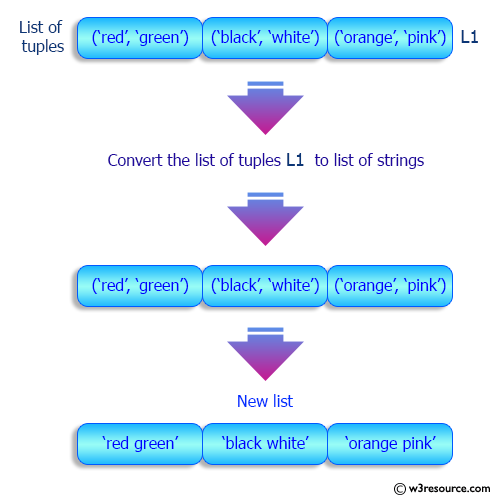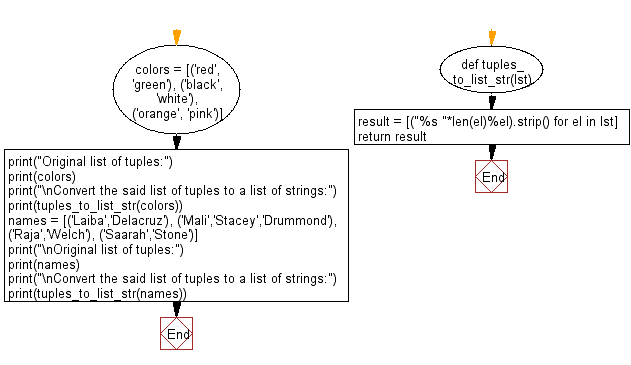﻿ Python: Convert a given list of tuples to a list of strings - w3resource# Python: Convert a given list of tuples to a list of strings

## Python List: Exercise - 187 with Solution

Write a Python program to convert a given list of tuples to a list of strings.

Sample Solution:

Python Code:

``````def tuples_to_list_str(lst):
result = [("%s "*len(el)%el).strip() for el in lst]
return result
colors = [('red', 'green'), ('black', 'white'), ('orange', 'pink')]
print("Original list of tuples:")
print(colors)
print("\nConvert the said list of tuples to a list of strings:")
print(tuples_to_list_str(colors))
names = [('Laiba','Delacruz'), ('Mali','Stacey','Drummond'), ('Raja','Welch'), ('Saarah','Stone')]
print("\nOriginal list of tuples:")
print(names)
print("\nConvert the said list of tuples to a list of strings:")
print(tuples_to_list_str(names))
```
```

Sample Output:

```Original list of tuples:
[('red', 'green'), ('black', 'white'), ('orange', 'pink')]

Convert the said list of tuples to a list of strings:
['red green', 'black white', 'orange pink']

Original list of tuples:
[('Laiba', 'Delacruz'), ('Mali', 'Stacey', 'Drummond'), ('Raja', 'Welch'), ('Saarah', 'Stone')]

Convert the said list of tuples to a list of strings:
['Laiba Delacruz', 'Mali Stacey Drummond', 'Raja Welch', 'Saarah Stone']
```

Pictorial Presentation:Flowchart:## Visualize Python code execution:

The following tool visualize what the computer is doing step-by-step as it executes the said program:

Python Code Editor:

Have another way to solve this solution? Contribute your code (and comments) through Disqus.

What is the difficulty level of this exercise?

Test your Python skills with w3resource's quiz

﻿

## Python: Tips of the Day

Floor Division:

When we speak of division we normally mean (/) float division operator, this will give a precise result in float format with decimals.

For a rounded integer result there is (//) floor division operator in Python. Floor division will only give integer results that are round numbers.

```print(1000 // 300)
print(1000 / 300)```

Output:

```3
3.3333333333333335```